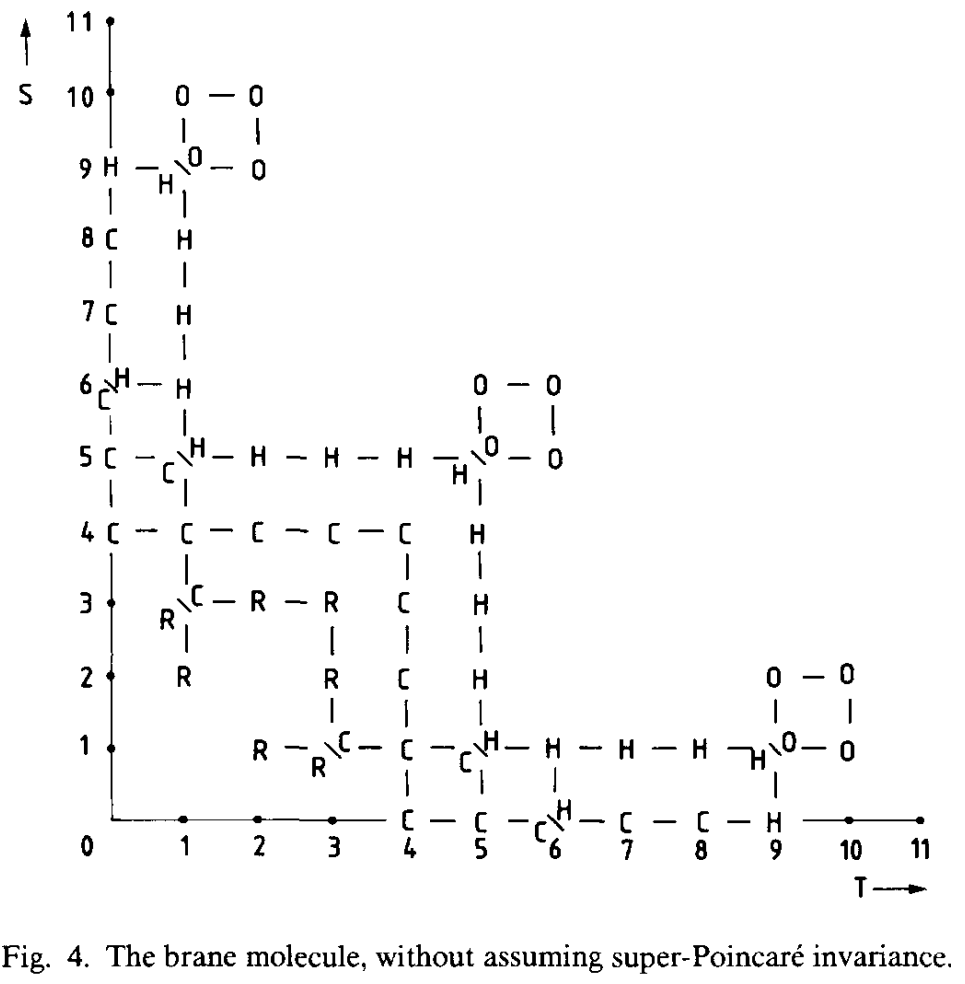Contents

supersymmetry

# Contents

## Idea

The brane scan of Green-Schwarz sigma-model for super p-branes shows a 4-brane in 9d.

The brane scan.

The Green-Schwarz type super $p$-brane sigma-models (see at table of branes for further links and see at The brane bouquet for the full classification):

$\stackrel{d}{=}$$p =$123456789
11M2M5
10D0F1, D1D2D3D4NS5, D5D6D7D8D9
9*
8*
7M2${}_{top}$
6F1${}_{little}$, S1${}_{sd}$S3
5*
4**
3*

(The first columns follow the exceptional spinors table.)

The corresponding exceptional super L-∞ algebra cocycles (schematically, without prefactors):

$\stackrel{d}{=}$$p =$123456789
11$\Psi^2 E^2$ on sIso(10,1)$\Psi^2 E^5 + \Psi^2 E^2 C_3$ on m2brane
10$\Psi^2 E^1$ on sIso(9,1)$B_2^2 + B_2 \Psi^2 + \Psi^2 E^2$ on StringIIA$\cdots$ on StringIIB$B_2^3 + B_2^2 \Psi^2 + B_2 \Psi^2 E^2 + \Psi^2 E^4$ on StringIIA$\Psi^2 E^5$ on sIso(9,1)$B_2^4 + \cdots + \Psi^2 E^6$ on StringIIA$\cdots$ on StringIIB$B_2^5 + \cdots + \Psi^2 E^8$ in StringIIA$\cdots$ on StringIIB
9$\Psi^2 E^4$ on sIso(8,1)
8$\Psi^2 E^3$ on sIso(7,1)
7$\Psi^2 E^2$ on sIso(6,1)
6$\Psi^2 E^1$ on sIso(5,1)$\Psi^2 E^3$ on sIso(5,1)
5$\Psi^2 E^2$ on sIso(4,1)
4$\Psi^2 E^1$ on sIso(3,1)$\Psi^2 E^2$ on sIso(3,1)
3$\Psi^2 E^1$ on sIso(2,1)

### The Brane molecule

Furthermore, there exists a more general classification of possible supermembranes in spacetime with $S$ spatial dimensions and $T$ time dimensions, appearing in (Blencowe-Duff 88). In this sense, the brane scan is but the $T=1$ branch of the brane molecule. The objects appearing here are expected to be related to other generalizations of string theory. See D=12 supergravity and bosonic M-theory.It’s generalization featuring L-infinity-algebra extensions is the brane bouquet.

Last revised on January 14, 2023 at 09:33:18. See the history of this page for a list of all contributions to it.